# Background

Let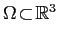be a bounded, simply or multiply connected domain in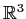with a Lipschitz boundary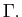The Poisson equation for a scalar function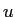in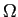is given byor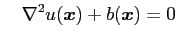or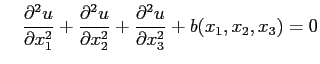whererepresents a source function prescribed on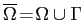.

A solutionof the Poisson equation admits an integral representation, known as the Green's representation formula, expressed as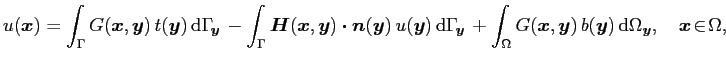where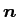is the unit normal to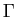directed towards the exterior of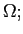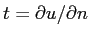is the flux associated with the function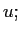and the kernels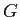and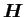are given respectively by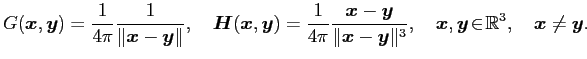Here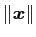is the usual Euclidean norm indefined as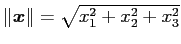. In addition, it was established in  that the Newton potential admits a boundary representation as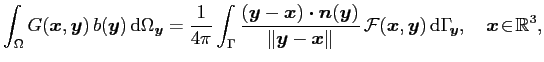where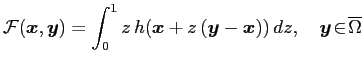with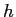denoting an extension of the source functioninto any ball centered at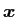and containing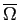. In particular, a continuationof the source functioncan be specified as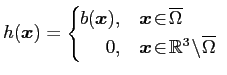This representation of the Newton potential in term of surface integral allows a numerical solution of the Poisson equation that does not require a volume-fitted mesh.

# Solution via a boundary element method

To approximately solve the Poisson equation via a Boundary Element Method (BEM), the surfaceis usually discretized into flat triangles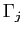using a mesh generation software (e.g. CUBIT).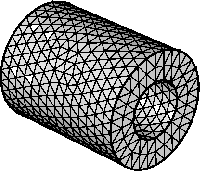With reference to , the functionsand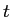are assumed to have a polynomial variation over each triangle (boundary element)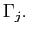Galerkin BEM

# References

 S. Nintcheu Fata.
Treatment of domain integrals in boundary element methods.
Appl. Num. Math., 62(6):720-735, 2012.